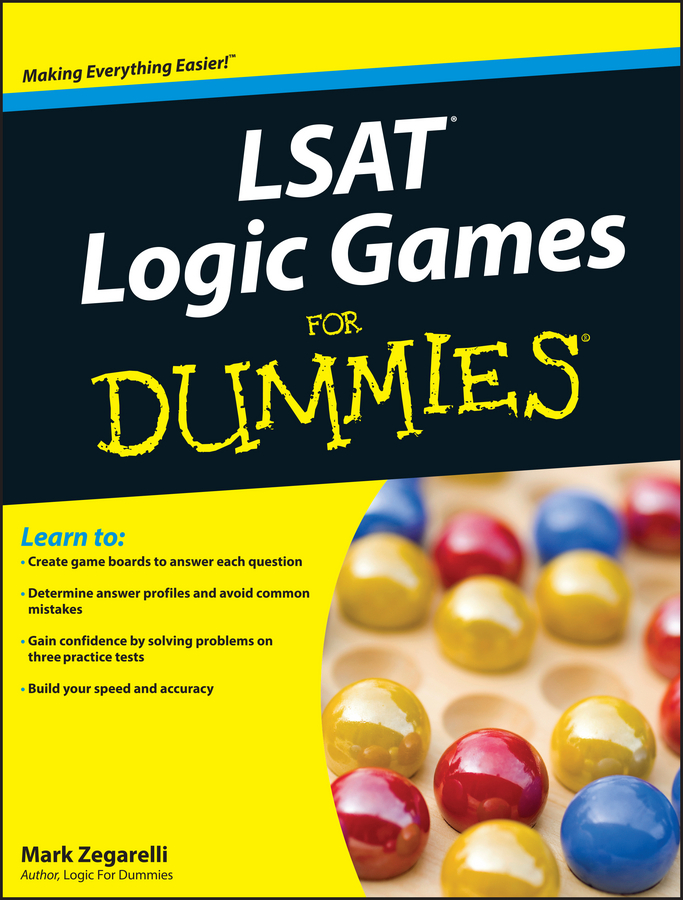##### LSAT Logic Games For DummiesWhen you factor, you break down an expression into the original terms that were (or could have been) multiplied to get that expression. When you take the PSAT/NMSQT, the factoring you have to do is pretty basic. You’ll likely see an expression with three terms that were calculated using FOIL. For example, you may have to factor x2 + 10x + 24 or something similar.

Here are the steps for factoring the example (x2 + 10x + 24):

• Draw two sets of parentheses. Now you have a “home” ready for two factors.

• Look at the first term and decide how you get there. The first term is x2, so you must have multiplied x by x. Place those values in the First spot in each parenthesis:

(x ) (x ).

• Examine the last term and decide how you get there. The last term is 24. To arrive at 24, you may have multiplied 24 by 1, 12 by 2, 8 by 3, or 6 by 4. Even two negative numbers work, because when you multiply two negatives, you get a positive number. Because you can’t tell, for the moment leave the Last spot in each parenthesis open.

• Look at the middle term. The numbers that you’re looking for to put into the parentheses along with the x’s need to add up to the value of the middle term. Of the aforementioned pairs, 24 and 1 give you 25 (too big), 12 and 2 give you 14 (too big), 8 and 3 give you 11 (still too big), and 6 and 4 give you 10 (perfect!).

Now you know that you need 6 and 4 in the spots: x2 + 10x + 24 = (x + 6)(x + 4). Keep in mind that if the numbers in the parentheses are negative, adding up may actually mean subtracting!

Just for practice, try this question:

1. The expression x2 – 2x – 15 is equivalent to

(A) x(x – 2)
(B) (x – 2)(x – 15)
(C) (x + 3)(x – 5)
(D) (x – 3)(x + 5)
(E) (x + 15)(x – 1)